You can't understand genetics without understanding DNA.

You can't understand asset allocation and investing without understanding the fundamental financial model used to creating portfolios of stocks.

That's the Markowitz model. Created in 1952, it was such a game-changer that Markowitz was awarded the Nobel Prize.

Understanding this model will give you a lot of clarity on a variety of important topics. You'll understand how mutual funds, ETFS and investment portfolios are created. No more black smoke and mirrors. You'll finally get it.

### The World of Finance

The world of multi-billion dollar hedge-funds and investing is a fascinating black box.

It's a game of hiring the most brilliant minds in mathematics, physics and computer science.

These minds, professionally called "quants", spend their time building sophisticated financial models and running thousands of simulations.

All in the search of finding opportunities to exploit and building an asset allocation strategy that makes a return sufficient enough to satisfy their investors.

But what is asset allocation? It's about picking stocks and other assets in the particular proportions to create your desired portfolio.

It's no different than cooking. To make the tastiest dishes, you need the right balance of spices.

To make a great portfolio, you need the right balance of assets.

In cooking you optimize for taste. In asset allocation, you optimize for a particular balance between risk and return.

Let's head back to the quants. They're sitting at their computers and building financial models to find "asset allocation" strategies.

But what are these strategies? And how do they come up with them?

#### The Only Variable that matters

When quants are building these strategies they often only look at one variable. Price.

When they're building their models they only care about the historical prices of several stocks. Not the competition in the industry, not how much revenue the company did, not the cost structure of the business.

This is called technical analysis. Which is fundamentally (no pun intended) different from fundamental analysis, which looks at balance sheets, income statements and the "fundamentals" of a company. Fundamental analysis is what investors like Warren Buffet use.

So how can these quants possibly build models with ONLY information about price?

We're about to dive into the Markowitz model, also referred to as Mean-Variance Optimization or Modern Portfolio theory.

Two concepts with big complex-sounding words, but this stuff is actually quite simple. Folks in academia and finance just like to name things so they sound important and difficult.

After reading this, you will have a strong mental model about how asset allocation works and how to solve the problem of creating a portfolio of stocks with a particular risk/rewards ratio in mind. .

## Key Rules

Before we introduce the Markowitz model, let's define some key rules and concepts.

1. When we're analyzing stocks, all we care about is historical prices.

2. Our goal is to create a portfolio that produces as much return as possible with as little risk as possible.

3. The process for achieving this goal is to first decide on the return we want. Then we let the computer run wild to find the asset allocation with the minimum risk that we can achieve for that return.

4. The approach to reaching this goal is to set what our desired return is and then set computers loose to find the minimal risk.

5. Return is measured by how much a stock or portfolio went up compared to the previous year. If a stock was \$100 and is now \$110, the return is 10%.

6. Risk is measured by something called variance. This concept is illustrated below.

Imagine that we've followed a stock for the past 5 years and tracked how much it's gone up or down in value each year.

So we have 5 numbers, each representing an annual rate of return.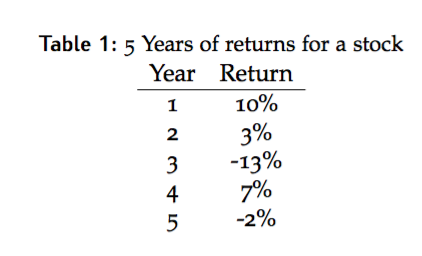The average of these returns is then calculated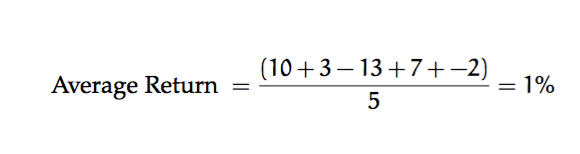We know the average of the returns is 1%.

But how certain are we that next year, the return will be 1% is? And if it isn't 1%, how far off will it be from 1%?

That's what variance measures.

So here's the formula for variance: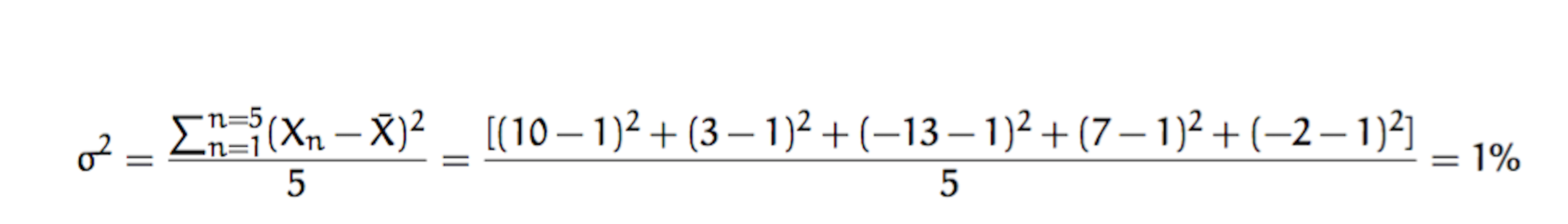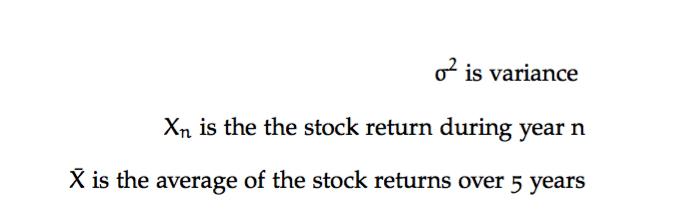The weird E looking thing is a summation operator. For each of the 5 years, we find out how far the return that year was away from the average and then we square that value. We sum up all these squares and then divide that by the total number of years. This gives us the average "spread" among all returns, the variance, which is 65.2%.

To recap, variance is uncertainty. Uncertainty is risk. If we could say with certainty that a stock price would never change, the variance would be 0%. The wider the swings in a stock price, the higher the variance.

7) The covariance of two stocks measure how they move and vary together.

If the covariance is positive it means that generally, when one stock moves up, so does the other and vice-versa. If the covariance is negative, it means that if one stock moves up, the other stock moves down and vice-versa.

Covariance is very similar to correlation and although there are mathematical differences, you can think of them as being conceptually the same.

Alright so those are the "laws". Now let's clearly define the problem and dive into the Nobel-prize winning Markowitz model

## Back to the goal

So we have \$10,000 and there are 4 stocks we're interested in (A,B,C,D).

Let's say that the return we'd like to aim for is 7%.

Our goal is to invest our \$10,000 in these 4 stocks so that we expect a return of at least 7% but also minimize our risk.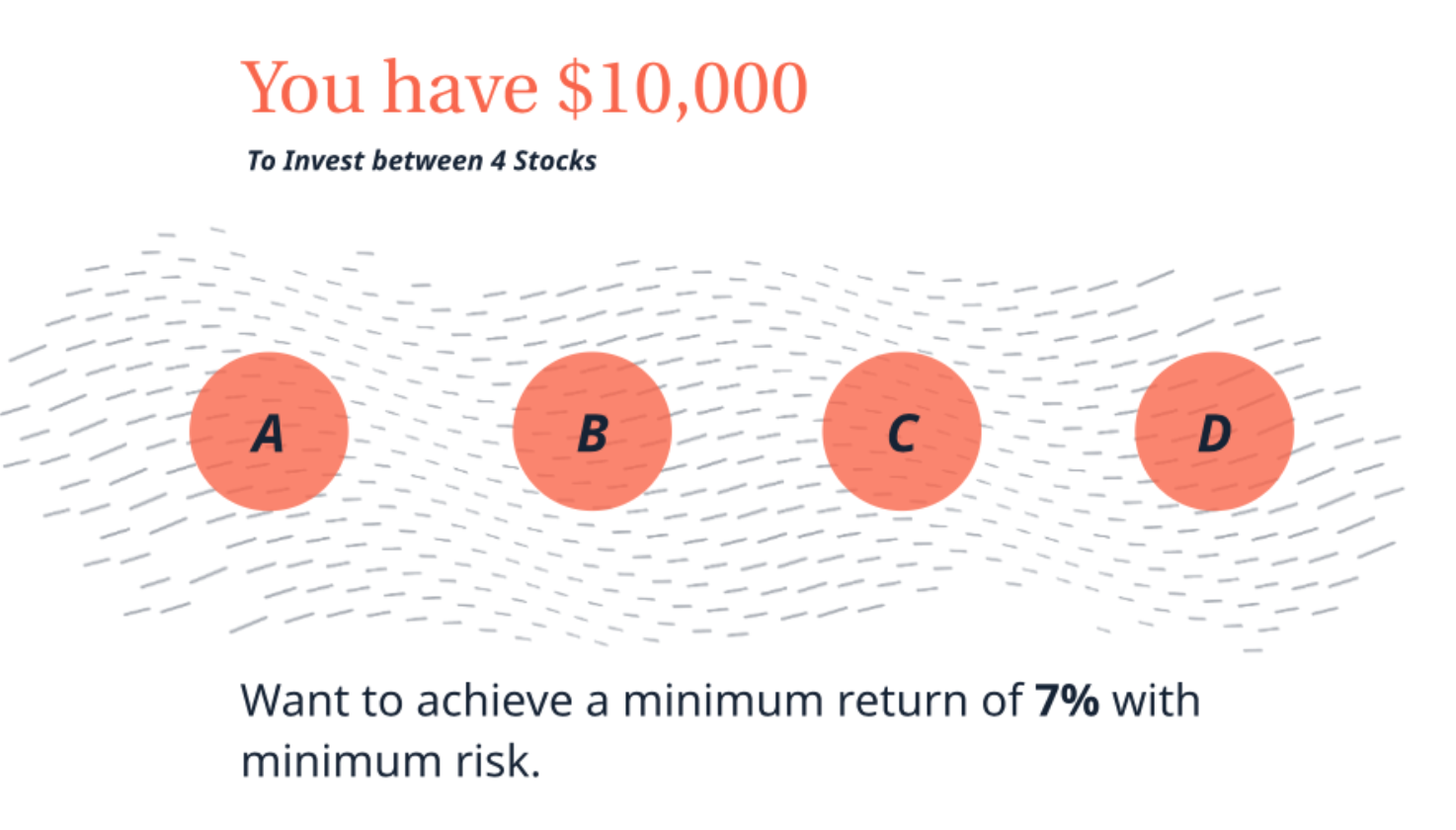We might typcially consider a few hundred or a few thousand. For simplicity's sake, let's assume that we're choosing between 5 stocks.

Here's a table that shows the returns for each of these stocks over the past 5 years.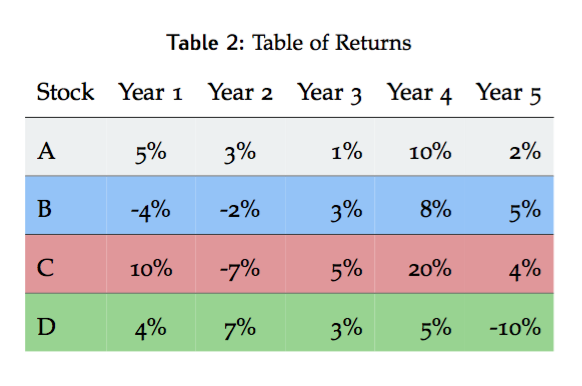So remember those concepts of correlation and covariance that we introduced earlier on? We're going to need to calculate them here. You can look up the math if you want to but it's quite similar to the way we calculated the variance earlier.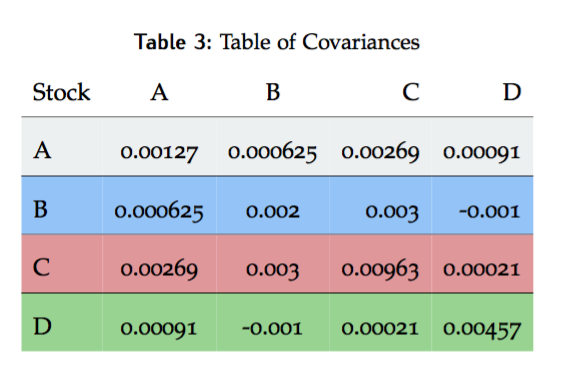Each number on the diagonal (A & A, B & B, etc.) represents the variance of that stock. Every other number represents how one stock moves in relation to the other, the covariance. For the most part, they all move in the same direction except for B & D, who generally move in opposite directions.

We previously only had price, but from the we were able to get return, variance and covariance. So that answers the question of why price data alone is enough!

Let's now check out this cool, curve-shaped graph.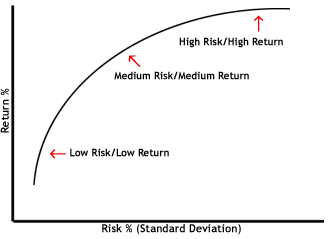Obviously, you can't just get a really high return without having a lot of risk. At some point, you reach a plateau. That's what this graph shows.

The graph is called an efficient frontier. If you were to place dots randomly on this graph, the dots above the curve would simply be impossible to reach (can't achieve that risk/return profile). The dots below the curve would be "inefficient". You could simply move up from the dot to the curve to get a higher return for the same amount of risk.

So we've been hyping up this amazing model that is the building block for investing and finance. Let's see exactly what it is.

## Markowitz Model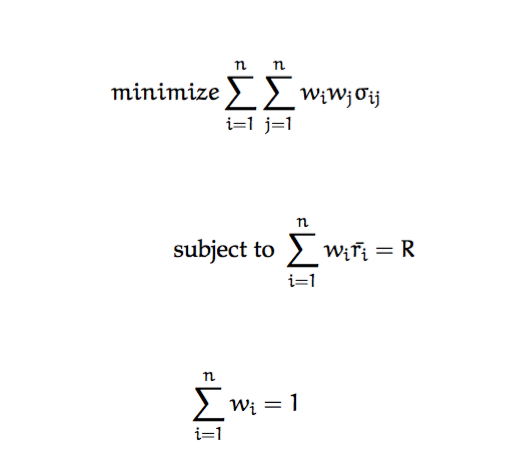And that's it, just 3 equations. But what does each mean? And what does the entire thing mean?

Let's define what each variable means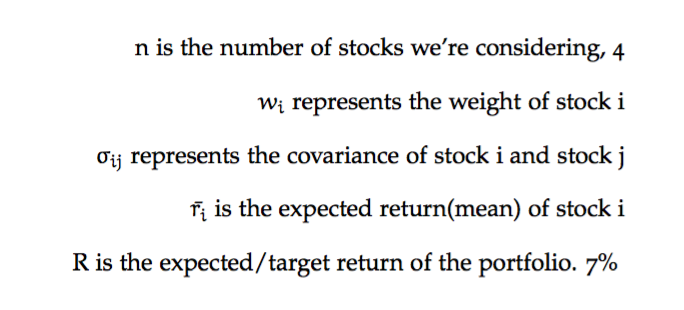The entire goal is to let the computer find the weights (or percentages) that each stock should contribute to the portfolio. That's what we're solving for.

Equation 1 is what's called the "objective function".

And the objective is to minimize the total varianc e(risk) of the portfolio.

The numbers on the right just show some of the math to calculate what the total variance is. It's calculating the covariance contributed to the portfolio by each pair of stocks and summing it up.

Equations 2 and 3 are constraints.

Equation 2 says that the sum of all stock weights has to equal 1. You can't have 3 stocks that take up 30%, 40% and 40% of a portfolio of course. That would be 110%! And you can't have it take up 90%. The stock weights have to account for 100% (or 1).

Equation 3 specifies what the minimum expected return of the portfolio should be. Expected return is just another name for mean or average. The way we calculate the average return of a portfolio is to sum up the average returns of each stock multiplied by the weight that each stock contributes to the portfolio.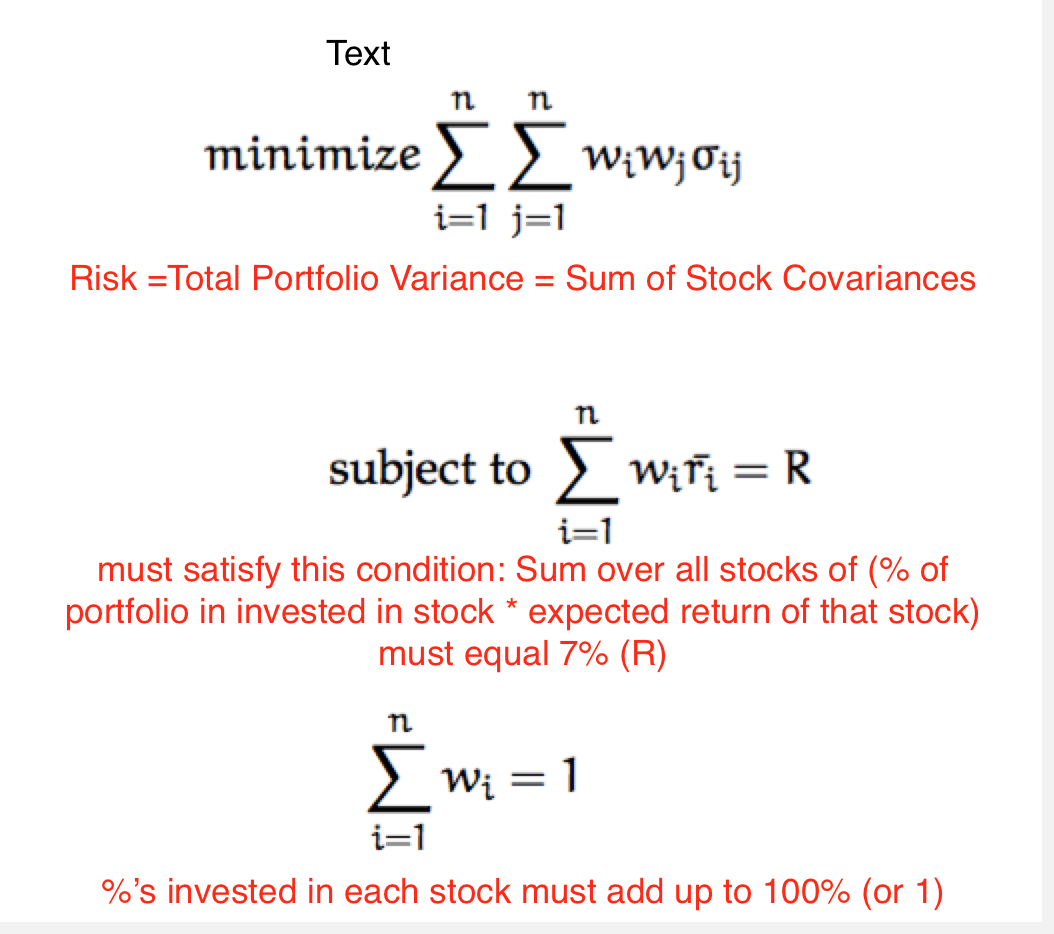Once we program these 3 equations into a computer and define the variables, the computer goes about trying to minimize the total variance of the portfolio while staying within the 2 specified constraints.

So now let's take a look at the results that the computer returned.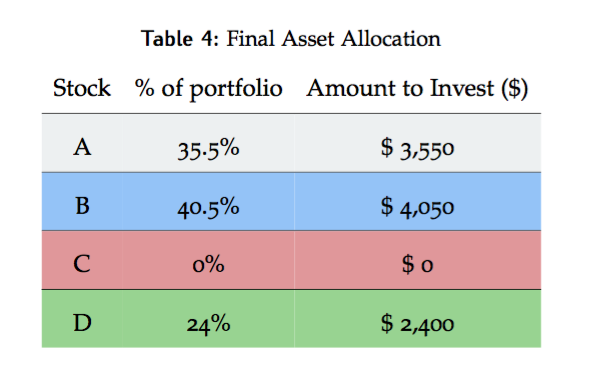And now we have an asset allocation strategy!

And you understand the most fundamental asset allocation used by investors.

But just because it's simple does not mean that Mean-Variance Optimization is a bad strategy. Quite the opposite!

I can't find any statistical analysis on this, but in the time I and colleagues have spent creating financial models, we found that in most cases, MVO outperforms them!

Sometimes the simplest things are the best!

## Recap

So here's a recap of what we've covered:

• Mean-Variance Optimization (also referred to as Modern Portfolio Theory) is the building block for asset allocation

• The goal of asset allocation is to get a particular

• The way we do this is by setting a target return and then finding the portfolio that minimizes the risk (the variance) for this particular return